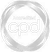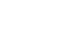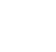# The Basics of Probability Distributions### Abdulla Javeri

In financial markets the concern is with earning returns. Although it is unknown what the actual returns will be, probability distributions allows investors to potentially quantify the various outcomes. In this first video of the series, Abdulla covers the very basics of probability distributions and constructs a simple example.

Financial MathsIn financial markets the concern is with earning returns. Although it is unknown what the actual returns will be, probability distributions allows investors to potentially quantify the various outcomes. In this first video of the series, Abdulla covers the very basics of probability distributions and constructs a simple example.

Speak to an expert

Speak to an expert today to access this and all of the content on our platform.### The Basics of Probability Distributions

4 mins 11 secs

Overview

Probability distributions can be used to predict an outcome for any type of experiment. The basics include a discussion of variables, setting up an experiment and recording results.

Key learning objectives:

• Learn the beginning stages of setting up a probability distribution

## Speak to an expert

Speak to an expert today to access this and all of the content on our platform.

Summary

#### What are random variables, experiments and outcomes?

Statisticians talk in terms of random variables, experiments and outcomes.

• A random variable is some sort of event that you’re looking at. For example, a flip of a coin, throw of a dice, the daily or weekly return on an asset, and so on.
• The experiment is actually observing one particular event, so one flip of the coin, one throw of the dice, the return for the chosen period.
• The outcome is the result of that experiment. Was it a head or a tail, was the dice throw a 1 or 2 etc., or what was the observed return for the period?

#### What is the difference between a discrete and continuous random variable?

Statisticians also talk in terms of discrete or continuous random variables.

A discrete variable is where there are definite or specific outcomes and there are intervals between those outcomes. Like for example a flip of a coin, it can only be heads or tails, the throw of a dice can only result in one of six specific outcomes with specific intervals. But if you look at something like daily returns, the intervals between outcomes could be so small as to be almost immeasurable, hence continuous. In other words, an infinite number of outcomes are possible and the probability of any one of those outcomes is virtually 0, but not 0.

#### What are the basics of probability distributions?

The basic principle of constructing a probability distribution is firstly conducting the experiments and getting the results or outcomes. The outcomes are then ranked from lowest to highest along with the frequency of each outcome. That gives us the frequency distribution. To work out the probability of each outcome simply divide the frequency of each outcome by the total number of outcomes. That converts the frequency distribution into the probability distribution.

## Speak to an expert

Speak to an expert today to access this and all of the content on our platform.### Abdulla Javeri

Abdulla’s career in the financial markets started in 1990 when he entered the trading floor of the London International Financial Futures Exchange, LIFFE, and qualified as a pit trader in equity and equity index options. In 1996, Abdulla became a trainer for regulatory qualifications and then for non-exam courses, primarily covering all major financial products.

There are no available videos from "Abdulla Javeri"

### Accredited by